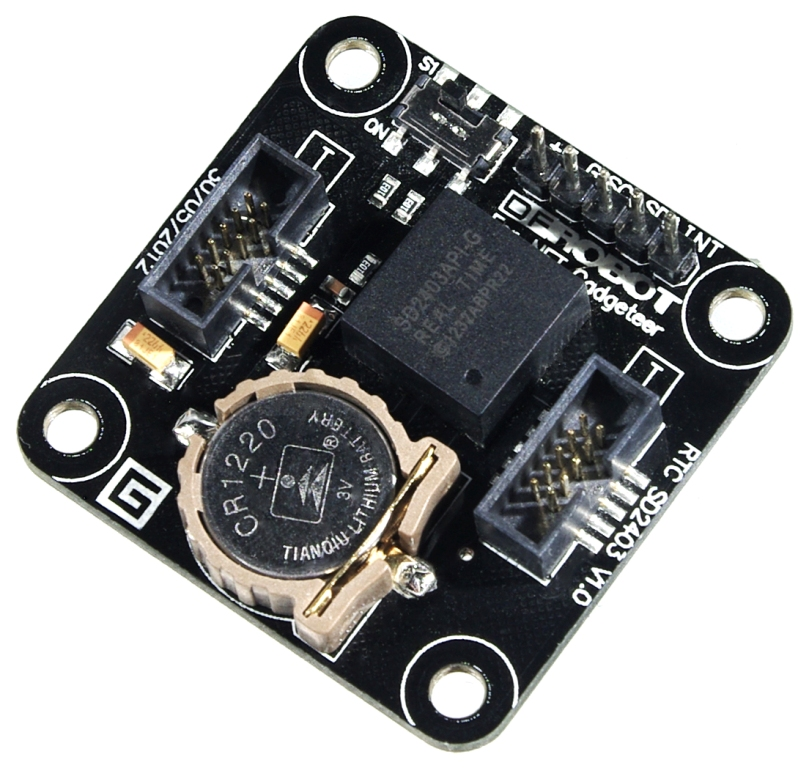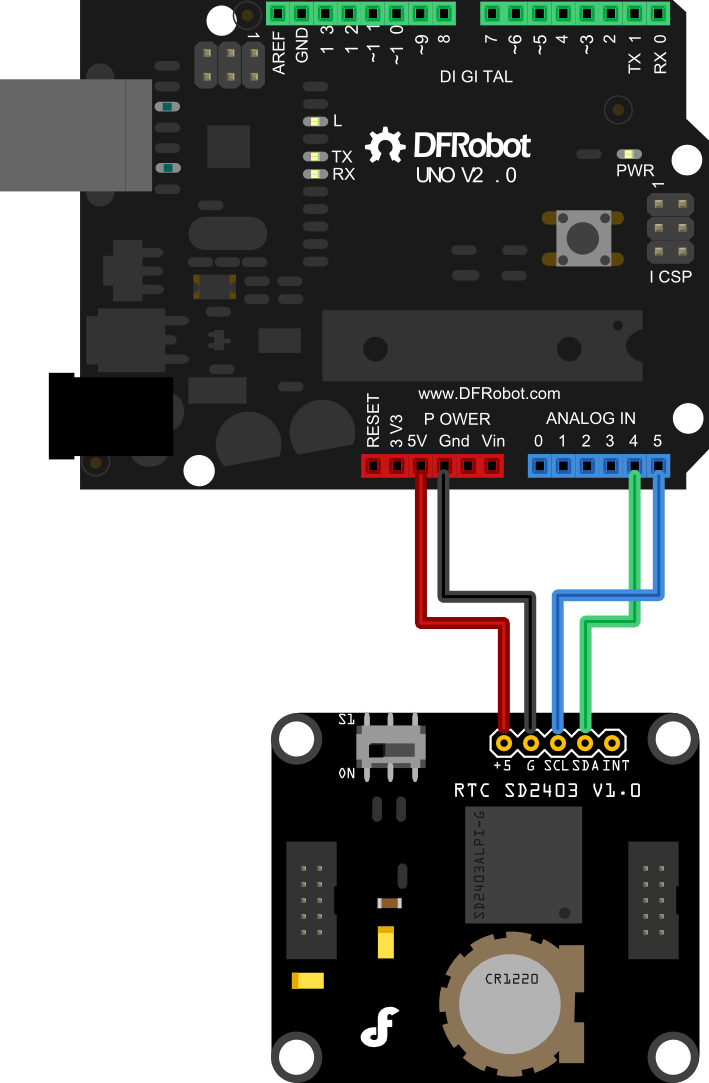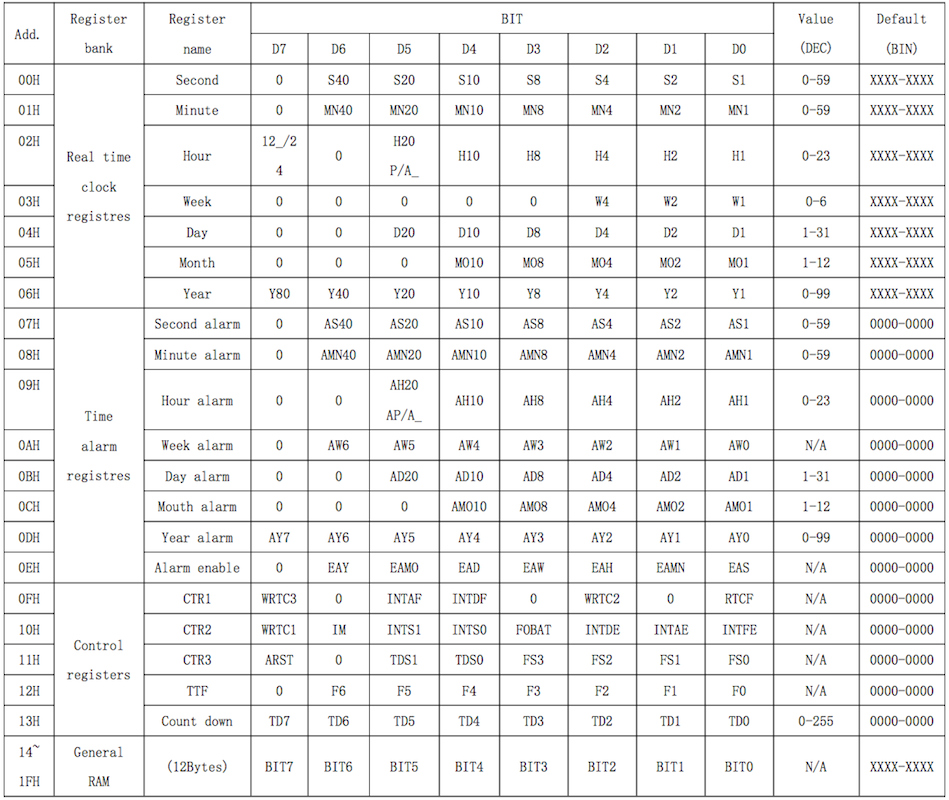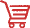# SD2403_Real_time_clock_Module__SKU_TOY0020_-DFRobot## IntroductionA real-time clock (RTC) is a computer clock (most often in the form of an integrated circuit) that keeps track of the current time.The term is used to avoid confusion with ordinary hardware clocks which are only signals that govern digital electronics, and do not count time in human units.

Compared with the cheap RTC module in the market now, this SD2403 Real-time clock module is low power consumption and high accurate solution. The typical power consumption is about 1.0uA. It intgerated the crystal and it ensure the accuracy of clock is about 5ppm.That means even if keep running one year, the deviation of this RTC module should be less than 2.5 minutes without the calibration. It supplies the time data via I2C interface and the time format is configurable to be switched between 12 hours and 24 hours. However, another good feature is the time alarm function via interrupt event output. So this RTC solution should be worth to try.

## Specification

• External power range: 1.8~5.5v
• Integrated with 3v battery socket
• Battery type: CR1220
• Support work without external power supply
• Supply fixed time alarm via interrupt output
• Typical power consumption: 1.1uA
• Selectable 12/24 hours format
• Interface: I2C and interrupt output
• Deviation: less than 2.5 minutes every year
• Pin ESD > 4KV
• 0.5s IIC Bus auto reset function to avoid from getting stuck
• Temperature range: -40~+85℃
• Switch for disconnecting the I2C pull-up resistors
• Size:36x36x14mm

## Connection Diagram## Example Code

``````#include <Wire.h>

//Pin Connection

unsigned char   date;

void setup()
{
Wire.begin();
Serial.begin(9600);
}

void loop()
{
I2CWriteDate();//Write the Real-time Clock
delay(100);

while(1)
{
Data_process();//Process the data

delay(1000);//延时1S
}
}

//Read the Real-time data register of SD2403
{
unsigned char n=0;

while(Wire.available())
{
}
delayMicroseconds(1);
Wire.endTransmission();
}

//Write the Real-time data register of SD2403
void I2CWriteDate(void)
{
WriteTimeOn();

Wire.write(0x59);//second:59
Wire.write(0x01);//minute:1
Wire.write(0x95);//hour:15:00(24-hour format)
Wire.write(0x03);//weekday:Wednesday
Wire.write(0x26);//day:26th
Wire.write(0x12);//month:December
Wire.write(0x12);//year:2012
Wire.endTransmission();

Wire.write(0x12);   //Set the address for writing
Wire.write(byte(0));
Wire.endTransmission();

WriteTimeOff();
}

//The program for allowing to write to SD2400
void WriteTimeOn(void)
{
Wire.write(0x10);//Set the address for writing as 10H
Wire.write(0x80);//Set WRTC1=1
Wire.endTransmission();

Wire.write(0x0F);//Set the address for writing as OFH
Wire.write(0x84);//Set WRTC2=1,WRTC3=1
Wire.endTransmission();
}

//The program for forbidding writing to SD2400
void WriteTimeOff(void)
{
Wire.write(0x0F);   //Set the address for writing as OFH
Wire.write(byte(0));//Set WRTC2=0,WRTC3=0
Wire.write(byte(0));//Set WRTC1=0
Wire.endTransmission();
}

//Process the time_data
void Data_process(void)
{
unsigned char i;

for(i=0;i<7;i++)
{
if(i!=2)
date[i]=(((date[i]&0xf0)>>4)*10)+(date[i]&0x0f);
else
{
date=(date&0x7f);
date=(((date&0xf0)>>4)*10)+(date&0x0f);
}
}
// Use the serial monitor to see information being transmitted
Serial.print("Sec = ");//second
Serial.print(date);
Serial.print("   Min = ");//minute
Serial.print(date);
Serial.print("   H = ");//hour
Serial.print(date);
Serial.print("   W = ");//week
Serial.print(date);
Serial.print("   D = ");//day
Serial.print(date);
Serial.print("   M = ");//month
Serial.print(date);
Serial.print("   Y = ");//year
Serial.print(date);

Serial.println();
}``````

## DataSheetThese RTC (Real time clock) registers are stored as binary-coded decimal BCD format.

Seconds and Minutes: range from 0 to 59;

Hour : can be set 12-hour or 24-hour mode;

day : from 1 to 31;

Month : from 1 to 12;

Year : from 0 to 99;

Day of the Week: from 0 to 6.

If 12_/24 bit of the Hour register is “1”, the RTC uses a 24-hour format. If the 12_/24 bit is “0”, the RTC uses a 12-hour format

For detail:Get *SD2403 Real time clock Modul SKU:TOY0020 * from DFRobot Store or DFRobot Distributor.